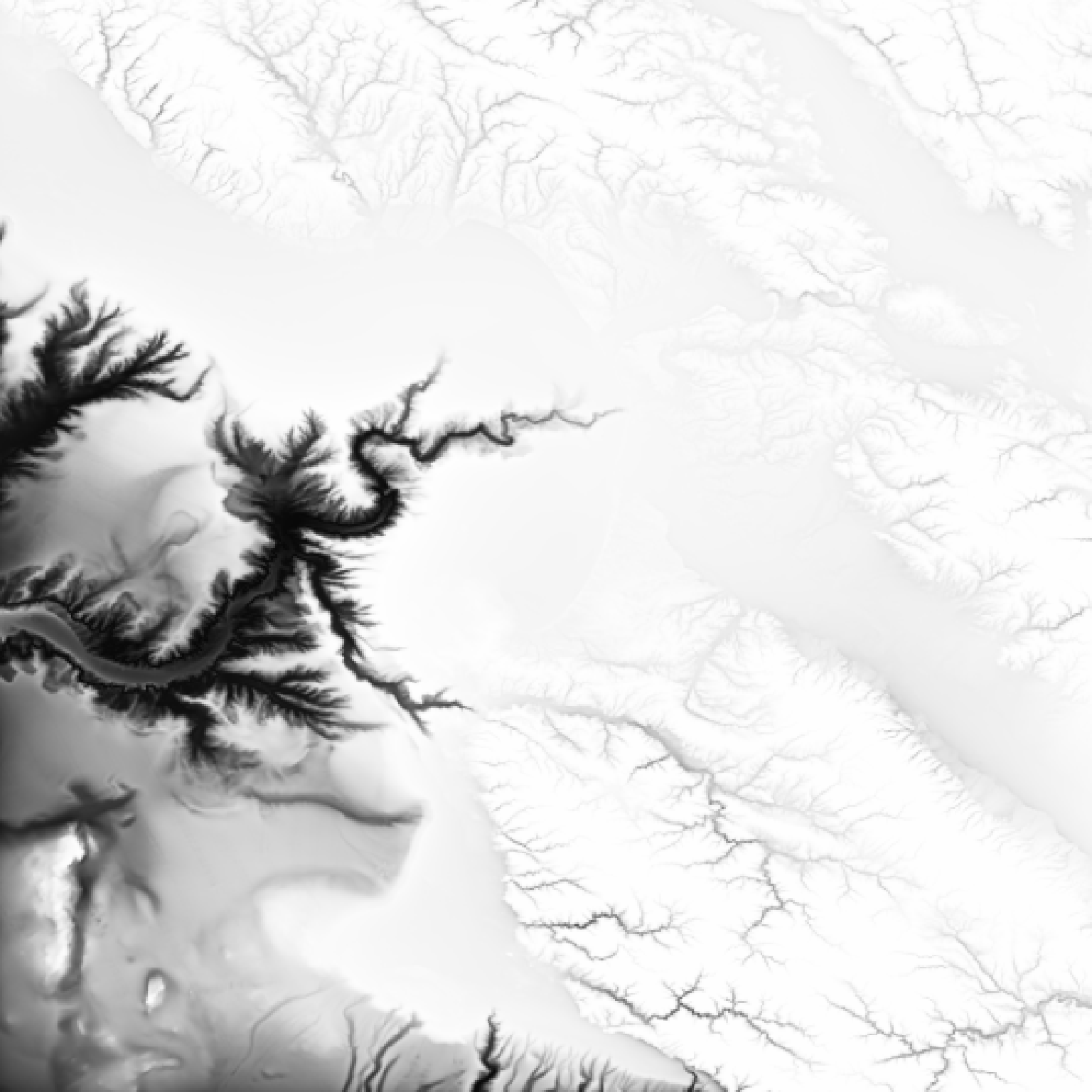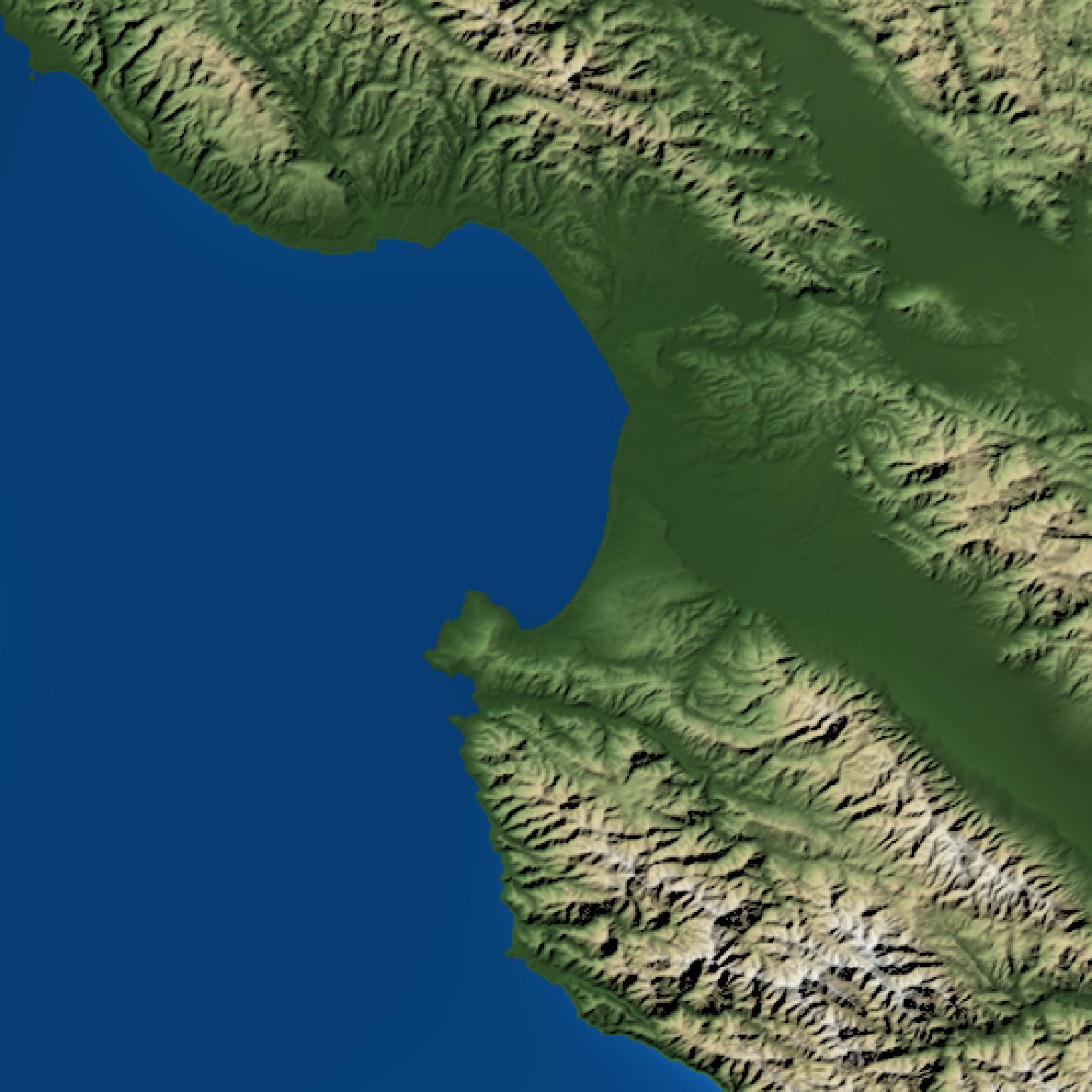Calculates a shadow for each point on the surface using the method described by Leland Brown in "Texture Shading: A New Technique for Depicting Terrain Relief."

texture_shade(
heightmap,
detail = 0.5,
contrast = 1,
brightness = 0,
transform = TRUE,
dx = 1,
dy = 1,
)

## Arguments

heightmap

A two-dimensional matrix, where each entry in the matrix is the elevation at that point.

detail

Default 0.5. Amount of detail in texture shading algorithm. 0 is the least detail, while 1 is the most.

contrast

Default 1,standard brightness. Amount of contrast in the texture shading. This transforms the resulting darkness using the formula tanh(input * contrast + brightness).

brightness

Default 0, standard brightness. Higher values will brighten the texture hillshade, while lower values will darken it.

transform

Default TRUE. Whether to apply the tanh(input * contrast + brightness) transformation. This transforms the resulting darkness using the formula tanh(input * contrast + brightness).

dx

Default 1. The distance between each row of data (compared to the height axis).

dy

Default 1. The distance between each column of data (compared to the height axis).

Default 50. The amount to pad the heightmap so edge effects don't appear from the fourier transform. Only increase this if you encounter boundary effects.

## Examples

#Create a direct mapping of elevation to color:
if(run_documentation()) {

#Plut using default values
montereybay %>%
plot_map()
}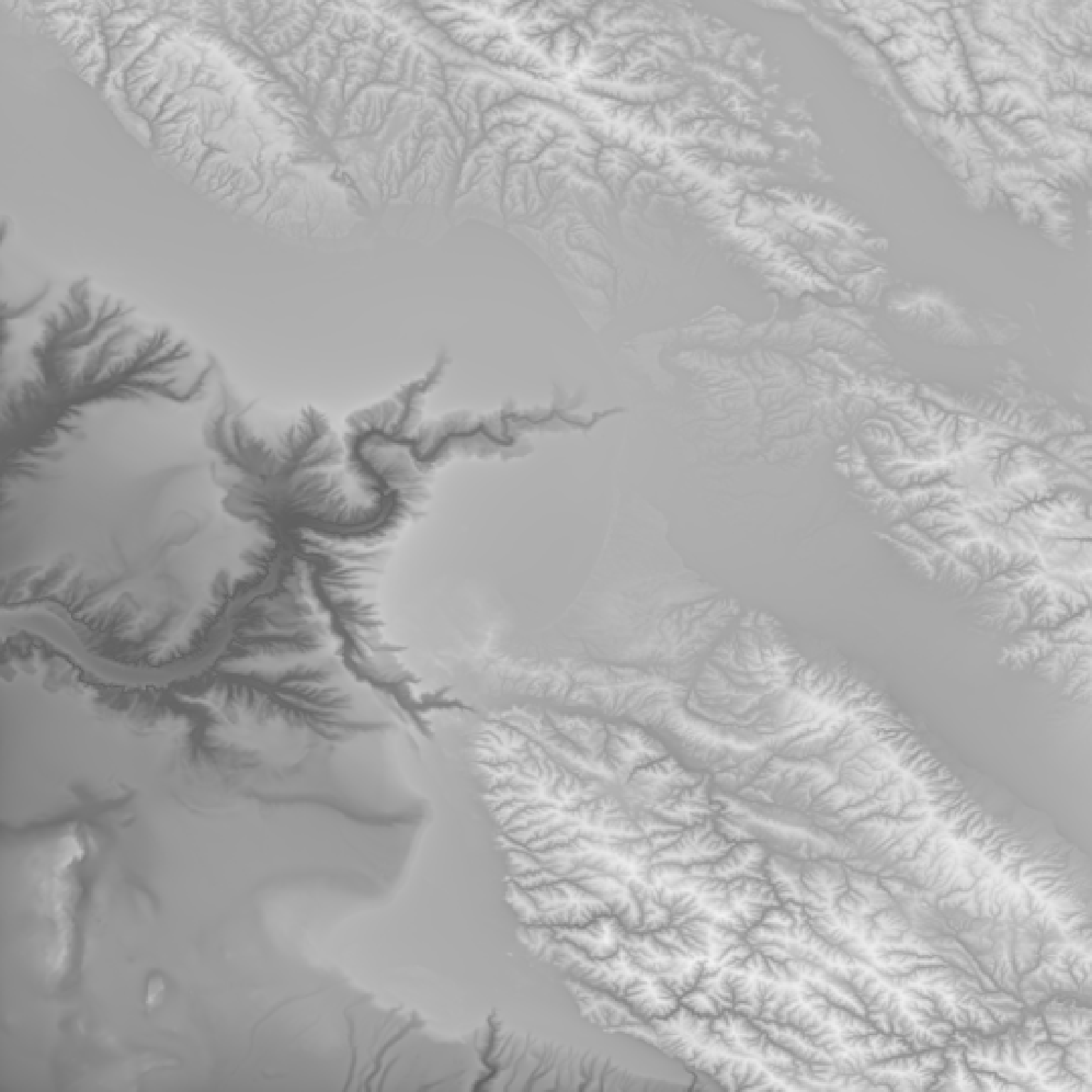if(run_documentation()) {
#Increase the level of detail
montereybay %>%
plot_map()
}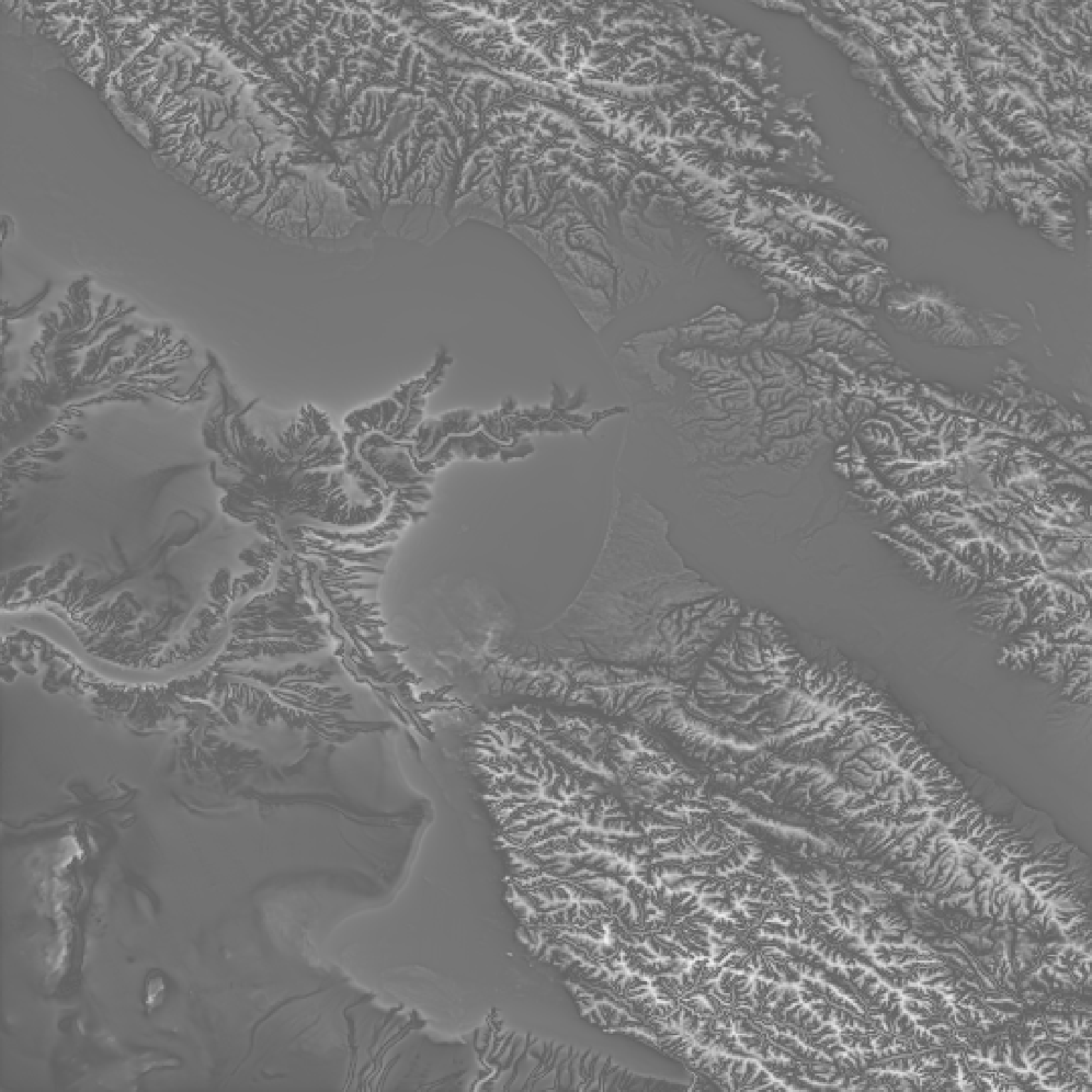if(run_documentation()) {
#Decrease the level of detail
montereybay %>%
plot_map()
}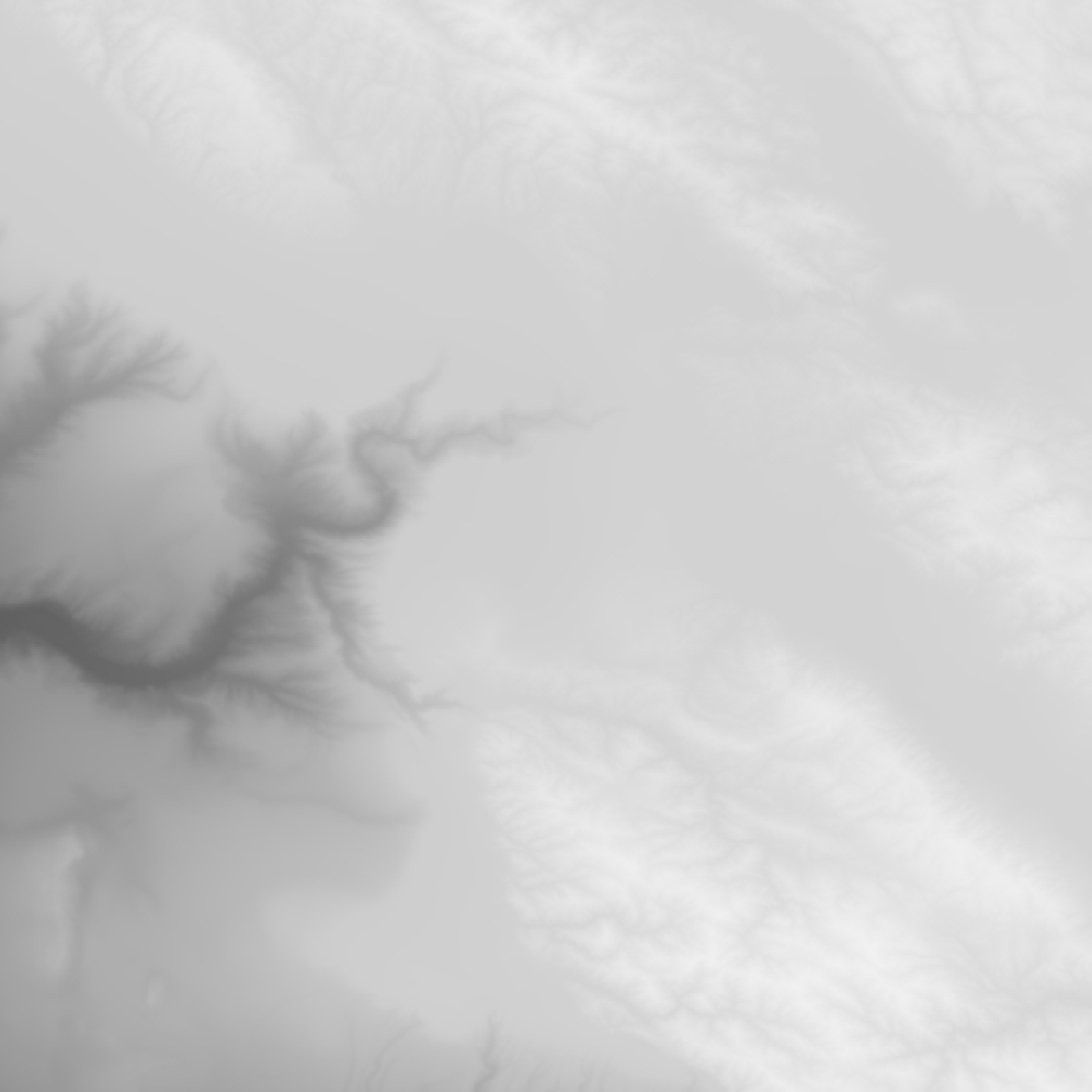if(run_documentation()) {
#Increase the level of contrast
montereybay %>%
plot_map()
}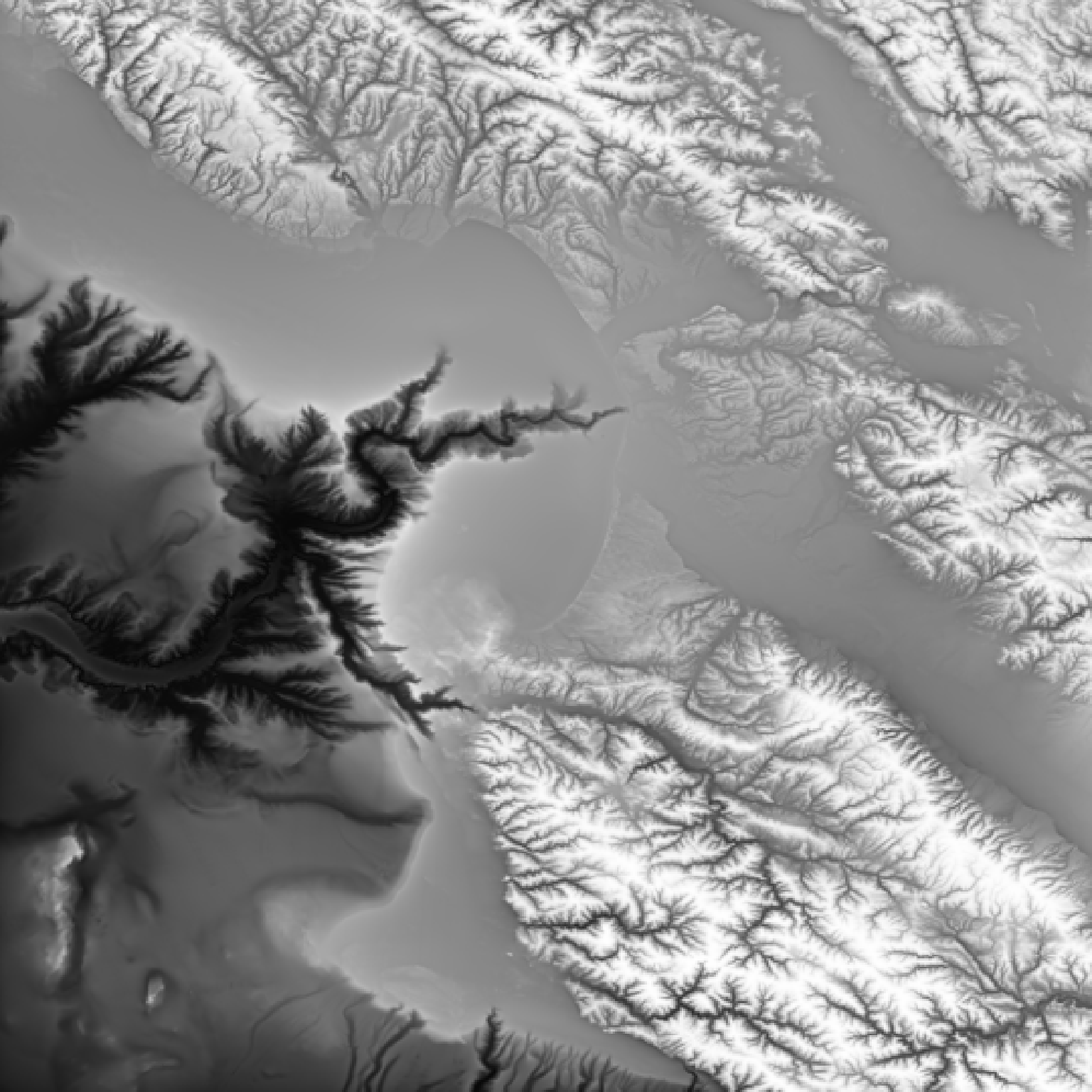if(run_documentation()) {
#Increase the brightness for this level of contrast
montereybay %>%
plot_map()
}